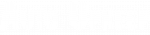# Resistance

An ohm (Ω) is a measure of electrical resistance. The resistance in a circuit is usually a load such as a light, radio, electrical motor, or sensor. For example, there needs to be resistance in the filament of a light bulb for it to produce light. Ohm’s Law shows the direct relationship between volts, amps, and ohms. Ohm’s law states Voltage = Current x Resistance. This simple mathematical equation can be used to find any unknown variable if the other two variables are known.